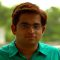Home » Core Java » Int to char Java ExampleVenkat works for a major IT firm in India and has more than a decade of experience working and managing Java projects for a banking client.

# Int to char Java Example

This article deals with the int to char Java conversion. We will start with the basics of data types and then we will see the conversion of int to char with some examples.

## 1. Data types in Java

Java is a strong statically typed language. This means that every variable and every expression has a type that is known at compile time. Also, the types limit the values that a variable can hold or an expression can produce. Strong static typing helps detect errors at compile time.

There are two kinds of types in Java – primitive types and reference types. Primitive type is predefined by Java and named by its reserved keyword. These can be either numeric or boolean type.

1. Numeric types are integral (byte, short, int, long, char) and floating point types (float and double).
2. Boolean type boolean represents a logical quantity with two possible values – true and false

Reference types can be class, interface and array.

The below table provides details about primitive data types:

 Data type Size in bits Range default value boolean – true, false false byte 8 -128 to 127 (inclusive) 0 short 16 -32,728 to -32,727 (inclusive) 0 char 16 ‘\u0000’ (or 0) to ‘\uffff’ (or 65,535 inclusive) ‘\u0000’ int 32 Signed: -2^31 to 2^31 -1Unsigned: 0 to 2^32 -1 0 long 64 Signed: -2^63 to 2^63 -1Unsigned: 0 to 2^64 -1 0 float 32 -2^-149 to (2 – 2^-23).2^127 0.0 double 64 -2^-1074 to (2 – 2^-52).2^1023 0.0

Refer the Java specs for more details.

## 2. ASCII character representation

ASCII is the acronym for American Standard Code for Information Interchange. The ASCII code associates an integer value for each symbol in character set, such as letters, digits, special characters. The ASCII table has 128 characters, with values from 0 through 127. Refer this link for details on each code.

ASCII values 0 through 31, and 127 represent non-printable control characters. The rest are printable. ASCII values 48 to 57 represent numbers from 0 to 9. ASCII values 65 to 90 represent A to Z and 97 to 122 represent a to z.

## 3. Conversion of primitive data types

Conversion of primitive data types in Java can be categorized as Widening primitive conversion and Narrowing primitive conversion.

Widening conversion is to convert a lower sized primitive data type to a higher sized type. This is an implicit conversion and would never result in a run-time exception. Example: int converted to a long, float or double (refer to the table above for size of each data type).

Narrowing conversion is to convert a higher sized primitive data type to a lower one. This may lose information about the overall magnitude of a numeric value and may also loose precision and range. Example: int converted to a byte, short or char

## 4. Int to char Java conversion

Let us now look into the conversion of int data type to char. This int to char conversion is narrow (refer the table above indicating the sizes). This would need a type cast as shown in example below.

The above code when executed would provide the result as below:

Value of c is :A
Non-printable ASCII. Value of c is :
Printable ASCII. Value of c is :3

We notice that when int value of 65 is converted to char, it gets converted to ASCII equivalent and prints value A. Similar observation can be made for 51. Conversion of int value 1 is non-printable.

## 5. Character.forDigit(int, int)

Character.forDigit(int digit, int radix) determines the character representation for a specific digit in the specified radix. It returns null if value of radix or digit is invalid. Refer java docs for further details.

radix can take value from 2 to 36 (both inclusive) and digit is valid if 0 <= digit < radix. Let us look at some examples

The above code when executed would give results as:

Conversion of digit 9 to radix 10:9
Conversion of digit 20 to radix 10:

Conversion of digit 10 to radix 16:a
Conversion of digit 20 to radix 16:

You would notice that the conversion returns null if either radix or digit is invalid.

In this article we discussed the conversions of int to char in java.

You can download the full source code of this example here: Int to char Java Example
0 0 vote
Article Rating

# Do you want to know how to develop your skillset to become a Java Rockstar?

## Subscribe to our newsletter to start Rocking right now!

### and many more ....

Subscribe
Notify of